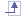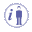# Mathematical Engineering Language (M-EKL)

This language gathers the language operators and the numerical functions (maths, measures, ...) necessary to express formulas and sets of equations used to valuate parameters provided in the Knowledge Advisor product.

• Formulas are objects integrated to the update process of CATIA V5. To be compliant with the update process, it is important that those objects manipulate only parameters as inputs or outputs (parameters being simple values, lists or geometric datums).
Formulas have only one parameter in output so that you cannot use functions that have arguments in output.
• Sets of equations rely on a mathematical solver and can only be used in conjunction with numerical parameters and mathematical functions.
 `y - 2 *sqrt(x) ==2;`## Knowledgeware and Extended Functions

Below is the list of operators and sets of functions that belong to M-EKL.

 Mathematical Functions Operators Analysis Operators Measures Part Measures
 Operators belonging to Knowledge products. Operators and constructors belonging to non Knowledge products.The information provided below constitutes the ground knowledge you must know to work with M-EKL.

## Constants

A constant is an identifier for a simple value. As the name suggests, this value cannot change. The following constants are specified or recognized by CATIA when programming rules and checks. As a result, they can be used anywhere in a relation in place of the actual values.

 `false` - one of the two values that a parameter of type Boolean can have `true` - one of the two values that a parameter of type Boolean can have `PI` - 3.141592  - The ratio of the circumference of a circle to its diameter. `E` - The base of natural logarithm - The constant e is approximately 2.718282.## Operators

### Arithmetic operators

`+  `Addition operator (also concatenates strings)

`-  `Subtraction operator

`*  `Multiplication operator

`/  `Division operator

( ) Parentheses (used to group operands in expressions)

`=  `Assignment  operator: Can be used with values, enumerates, objects (object A = object B if B is a sub-type of A)

**   Exponentiation operator

### Logical Operators

and   Logical conjunction on two expressions

or    Logical disjunction on two expressions

### Comparison Operators

<>   Not equal to (Applies to values and objects)

==   Equal to (Applies to values and objects)

>=   Greater or equal to (Applies to values only)

<=   Less than or equal to (Applies to values only)

<    Less than (Applies to values only)

>    Greater than (Applies to values only)## Units

Units are provided in the dictionary.

1. Pay attention to unit consistency.

2. PUnits are written with an underscore instead of the usual "/"  (example N_m2 instead of N/m2).

The /*  and */ comment characters are supported.

``````/* Set of Equations created by CRE 05/03/05 */
``````

## Others

When working with strings:

• If you want to insert quotation marks ("") inside quotation marks, use the \ special character.
 ```Message("you want to insert \" in your text.")```
• If you want to insert a back slash inside quotation marks, enter \\.
 `Message("xx\\yy")`